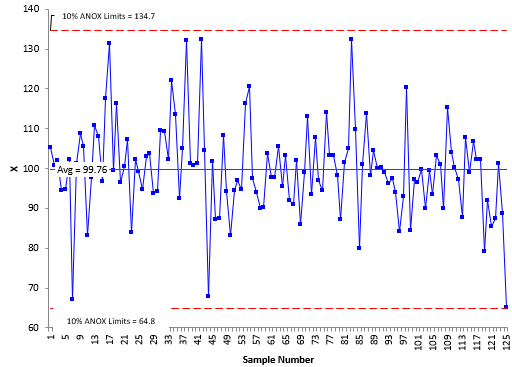## What is the Analysis of Individual Values (ANOX)

You have just finished a pilot production run for a new product. You want to know if the data you have are homogeneous, i.e., they come from one process and there are no outliers. How do you do that? In the past, I simply put the data on an individuals (X-mR) control chart. If the chart was in statistical control, I would assume that the data were homogeneous.

That changed when I read a 2017 article by Dr. Donald Wheeler and James Beagle III that described a new test for homogeneity. The problem with using a control chart to do a test for homogeneity is that the number of data points set the alpha level, which is the risk of a false alarm.  This is different from “one-time” tests where you pick the risk of a false alarm. For example, in determining if the means of two processes are the same, you might select an alpha = 0.05. You can’t pick the alpha level with the control chart approach – it is set by the number of points used to set the control limits.

Suppose you have k individual values. You would like to know if the data are homogeneous. The ANOX approach is given by the following steps:

• Calculate the moving range for each successive difference between the individual values
• Calculate the average moving range
• Calculate the average of the k individual values
• Determine the alpha value you want to use (risk of false alarm)
• Determine the scaling factor ANOXα (depends on alpha and the number of data points)
• Calculate the upper and lower ANOX limits: average ± ANOXα(average moving range)
• Interpret the chart for homogeneity

An example of an ANOX chart is given below. It does look a lot like a control chart – the difference is that the ANOX limits depend on alpha and the number of data points used. Typically, alpha is 1%, 5% or 10%. As long as the points are within the ANOX limits, the data are assumed to be homogenous – which is true in this example.For more information, please see our SPC Knowledge Base article, Analysis of Individual Values (ANOX). You can download a table of the scaling factors in that article as well. Our SPC for Excel software also contains the Analysis of Individual Values technique.

### Filtered HTML

• Allowed HTML tags: <a> <em> <strong> <cite> <code> <ul> <ol> <li> <dl> <dt> <dd> <h1> <h2> <h3> <h4> <h5> <h6> <img> <hr> <div> <span> <strike> <b> <i> <u> <table> <tbody> <tr> <td> <th>
• Lines and paragraphs break automatically.

### Plain text

• No HTML tags allowed.
• Lines and paragraphs break automatically.
This question is for testing whether you are a human visitor and to prevent automated spam submissions.

#### SPC Knowledge BaseSign up for our FREE monthly publication featuring SPC techniques and other statistical topics.